# # 3 数据流分析-应用

## # 3.1 数据流分析初步

### # 3.1.1 基本概念

• 分析的对象是基于抽象（Abstraction，见定义1.7）的 应用特定型数据（Application-Specific Data）

• 分析的行为是数据的“流动”，分析的方式是 安全近似（Safe-Approximation） ——根据安全性需求选择过近似（Over-Approximation）还是欠近似（Under-Approximation）；

• 分析的基础是控制流图（Control Flow Graph, CFG），CFG是程序 $P$ 的表示方法；

• 数据流动的场景有两个：

• 在CFG的点（Node）内流动，即程序基块（Basic Block，见定义2.2）内部的数据流；

• 在CFG的边（Edge）上流动，即由基块间控制流触发的数据流。

• 不同的数据抽象（Data Abstraction）;

• 不同的流安全近似策略——过近似或者欠近似；

• 不同的转移函数（Transfer Function，见定义1.8）和控制流处理方法（Control-Flow Handlings）。

• 称每一个 IR 语句 $s$ 执行之前 $pre(s)$ 执行之后的数据流值为 $s$输入状态（Input State） ，记为 $IN[s]$

• 其中， $pre(s)$ 为控制流中 $s$ 的前驱的集合（Predecessors，见定义2.4）；
• 称每一个 IR 语句 $s$ 执行之后 $suc(s)$ 执行之前的数据流值为 $s$输出状态（Output State） ，记为 $OUT[s]$

• 其中， $suc(s)$ 为控制流中 $s$ 的后继的集合（Successors，见定义2.4）；

$PP = \{(s_i, s_j) | s_i, s_j\in S \wedge (s_j \in suc(s_i) \vee s_i \in pre(s_j))\}$

$IN[s] = \bigwedge_{s_i \in pre(s)} OUT[s_i]$

$OUT[s] = \bigwedge_{s_i \in suc(s)} IN[s_i]$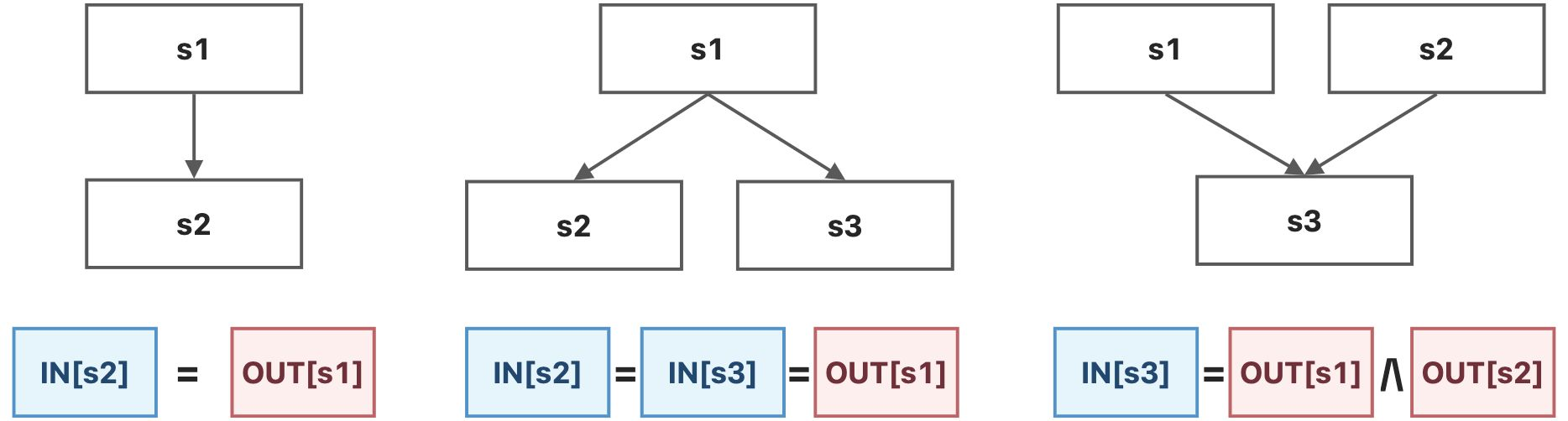• 基于语句语意（Sematics of Statements）的约束，即由状态转移方程（见定义3.7）产生的约束；

• 基于控制流（Flow of Control）的约束，即定理3.2所产生的约束。

### # 3.1.2 约束记号

$\forall i = 1, 2, ..., n - 1, IN[s_{i + 1}] = OUT[s_i]$

$OUT[B] = f_B(IN[B])$

$IN[B] = f_B(OUT[B])$

## # 3.2 定义可达性分析

### # 3.2.1 问题简化

• 方法调用（Method Calls）

• 我们只考虑过程内部（Intra-Procedural）的CFG；

• 跨过程分析（Inter-Procedural Analysis）将在之后的章节中展开。

• 别名（Aliases）

• 在我们之后的分析中，每个变量都有唯一的一个名字，没有别名；

• 关于别名的分析将在指针分析（Pointer Analysis）相关的章节中展开。

### # 3.2.2 问题描述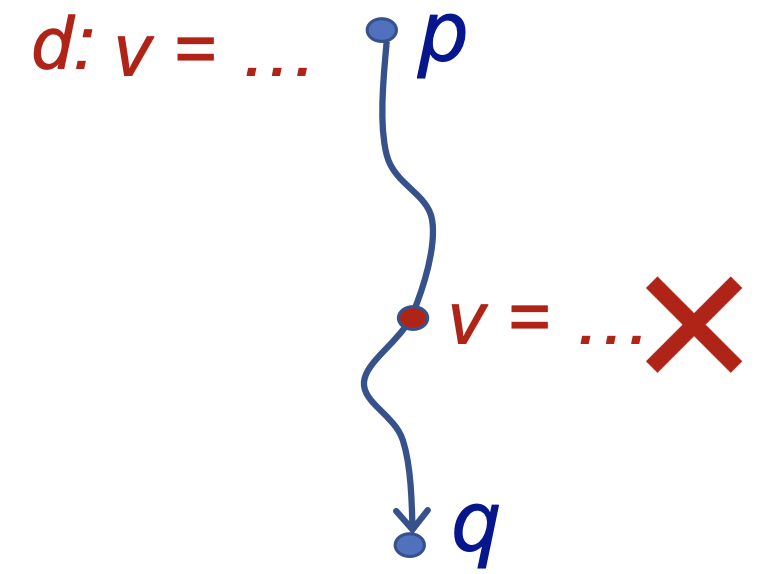### # 3.2.3 问题分析

#### # 约束分析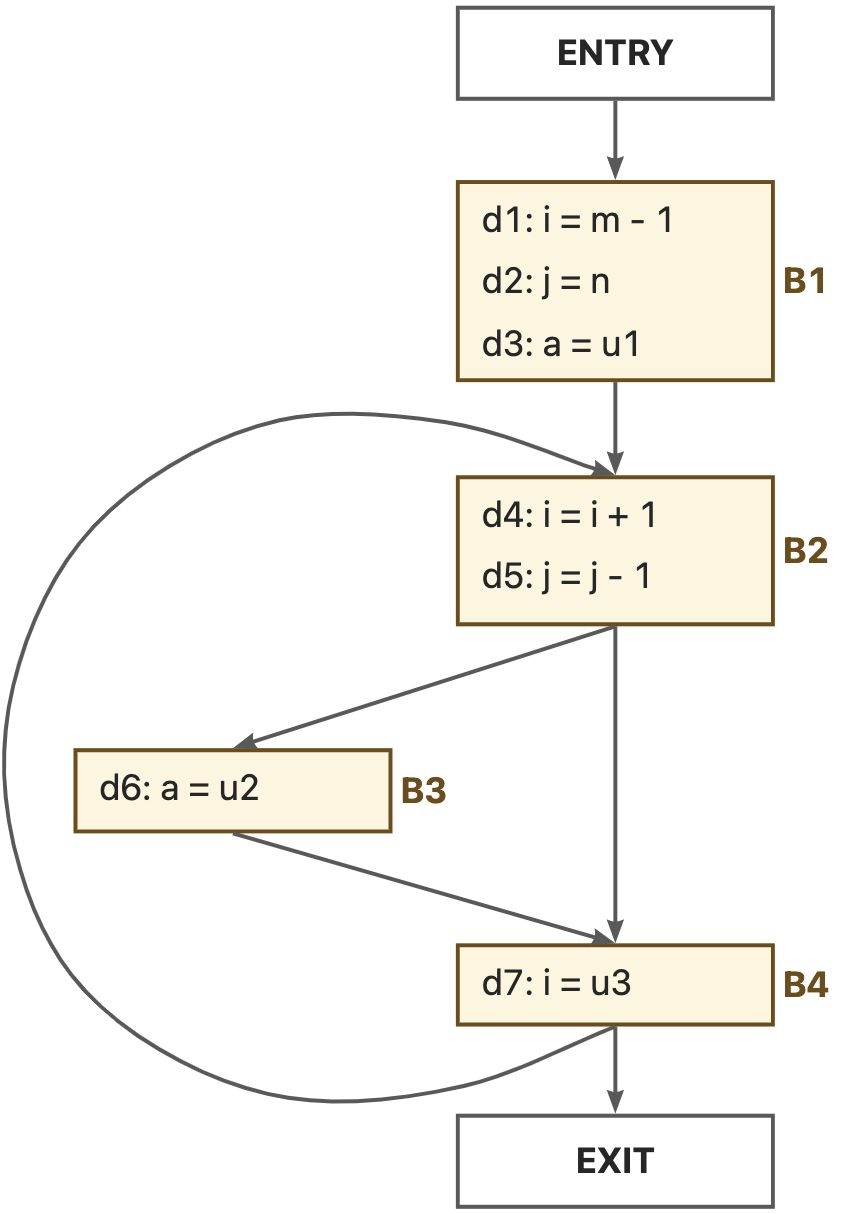$gen_{B_1} = \{d_1, d_2, d_3\}, kill_{B_1} = \{d_4, d_5, d_6, d_7\}$
$gen_{B_2} = \{d_4, d_5\}, kill_{B_2} = \{d_1, d_2, d_7\}$
$gen_{B_3} = \{d_6\}, kill_{B_3} = \{d_3\}$
$gen_{B_4} = \{d_7\}, kill_{B_4} = \{d_1, d_4\}$

$OUT[B] = gen_B \cup (IN[B] - kill_B)$

$IN[B] = \bigcup_{P \in pre(B)} OUT[P]$

### # 3.2.4 问题求解

#### # 算法设计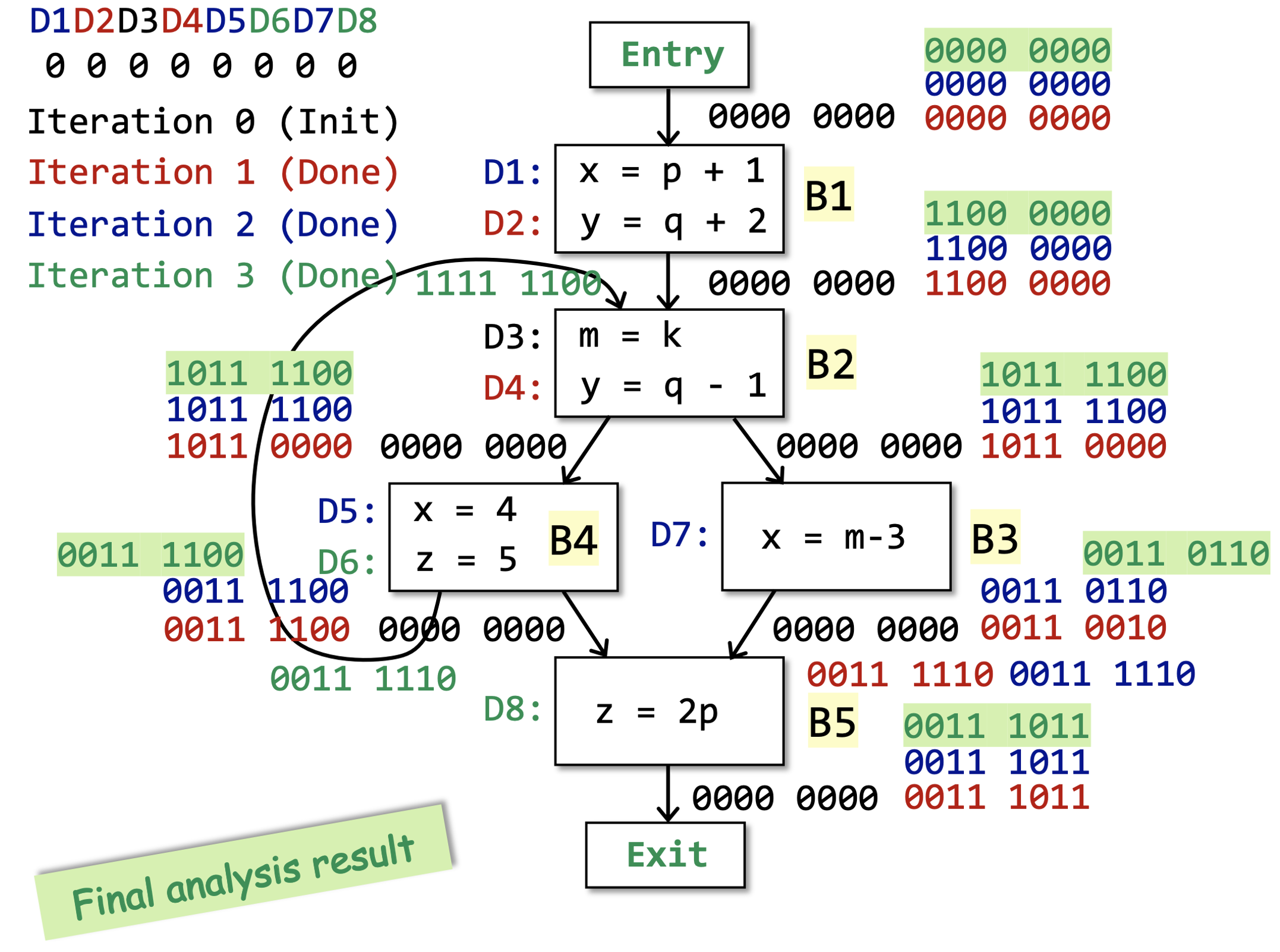#### # 算法分析

• $gen_B$$kill_B$ 是不变的，因为程序P本身是不改变的（至少我们现在的分析场景下是这样的）；

• 当更多的定义从控制流流入 $IN[B]$ （也就是当别处的定义到达B的时候），这里流入的更多的定义

• 要么被 $kill_B$ 给覆盖了，

• 要么幸存了下来，流入了 $OUT[B]$ ，记为 $survivor_B = IN[B] - kill_B$

• 也就是说，当一个定义d被加入 $OUT[B]$ 集合的时候，无论是通过 $gen_B$ 还是 $survivor_B$ ，它会永远的留在 $OUT[B]$ 中；

• 因为这一轮的幸存者在下一轮依然是幸存者（ $kill_B$ 是固定的）。
• 因此，集合 $OUT[B]$ 是不会收缩的，也就是说 $OUT[B]$ 要么变大，要么不变。

• 而定义的总集合 $D$ 是固定的，而 $OUT[B] \subseteq D$ ，因此最终一定会有一个所有的 $OUT[B]$ 都不变的状态。

• 最多的迭代次数为 $|D| \times |\{B | B\ is\ a\ basic\ block\ of\ P\}|$ ，因为最慢的时候就是每次迭代只有一个 $OUT[B]$ 增加了一个元素。

## # 3.3 活跃变量分析

### # 3.3.1 问题描述### # 3.3.2 问题分析

#### # 约束分析

• 第一种是 $OUT[B]$ 中已经存活，并且在$B$并没有重定义；

• 第二种是在 $B$ 中被使用的在 $B$ 之前定义的变量（也就是说，如果 $B$ 自己先定义了一个变量，然后再使用这个变量，那么这个变量在 $IN[B]$ 处还是死的）。$IN[B] = use_B \cup (OUT[B] - def_B)$

$OUT[B] = \bigcup_{S \in suc(B)} IN[S]$

### # 3.3.3 问题求解

#### # 算法设计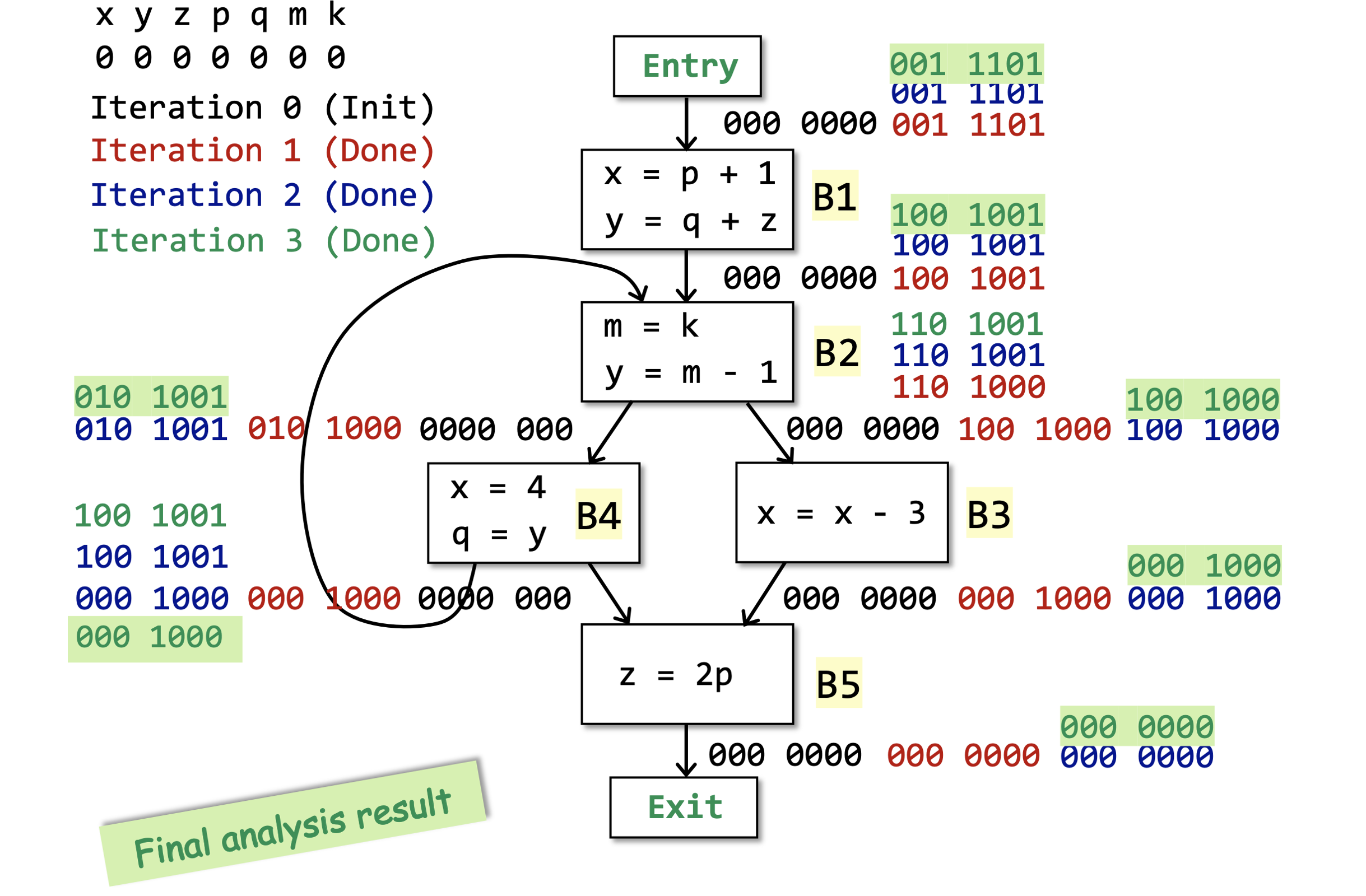#### # 算法分析

• 分析顺序，3.1是顺序分析，3.2是逆序分析；

• 初始化的时候，3.1先初始化的是输出状态，而3.2先初始化的是输入状态，其实也是由分析顺序决定的。

## # 3.4 可用表达式分析

### # 3.4.1 问题描述

• 所有 的从程序入口到程序点 $p$ 的路径都 必须 经过 x op y 表达式的评估（Evaluation），并且

• 在最后一次 x op y 的评估之后，没有 $x$ 或者 $y$ 的重定义（Redefinition）。

if a - b > c then
c = a - b;

1
2
d = a - b;
if d > c then
c = d

1
2
3

### # 3.4.2 问题分析

#### # 约束分析

$OUT[B] = gen_B \cup (IN[B] - kill_B)$

$IN[B] = \bigcap_{P \in pre(B)} OUT[P]$

### # 3.4.3 问题求解

#### # 算法设计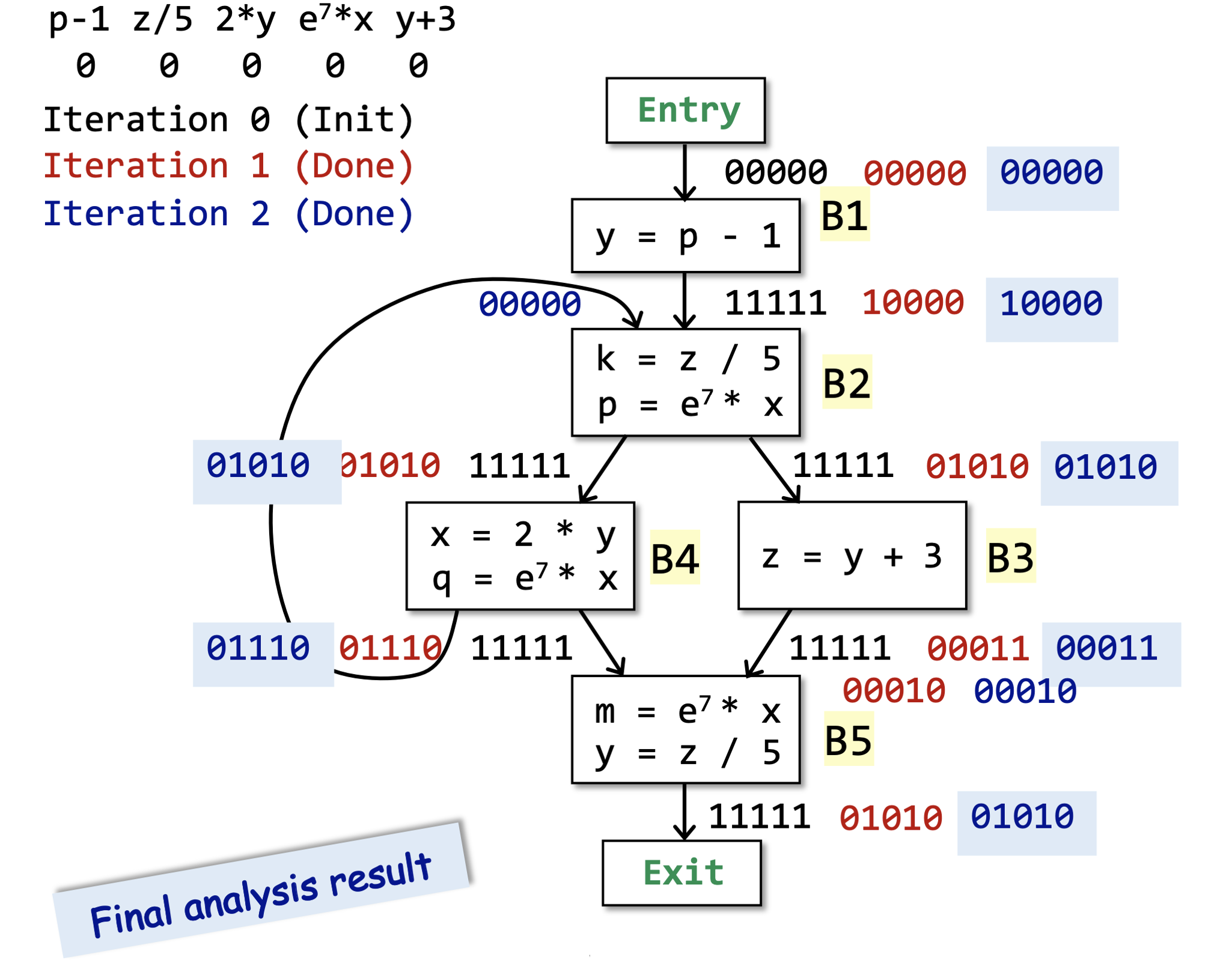#### # 算法分析

• 初始化的时候，除了程序入口之外的其他基块B的 $OUT[B]$ 都初始化为了全集，因为在最开始的时候，所有的表达式都是可用的。只有当我们在分析过程中发现表达式中的某个变量被重定义的时候，表达式才会变得不可用（需要重新计算）。

• 控制流约束部分变成了交集，因为我们这里是必然性分析。

## # 3.5 总结

1. 问题描述：

• 定义要研究的问题，从而确定分析顺序（正向还是逆向）和估计方式（过近似还是欠近似）；
2. 数据抽象：

• 确定抽象数据状态集（也就是数据流值集），从而确定定义域；
3. 约束分析：

• 考虑语意约束，确定状态转移方程；

• 考虑控制流约束，确定交汇操作符的含义；

4. 算法设计：

• 根据上述分析设计算法，我们目前只学了迭代算法，还可以有其他的算法设计；
5. 算法分析：

• 分析算法的正确性和复杂度。

## # 3.6 自检问题

1. 定义可达性（Reaching Definitions）分析、活跃变量（Live Variables）分析和可用表达式（Avaliable Expressions）分析分别是什么含义？

2. 上述三种数据流分析（Data Flow Analysis）有哪些不同点？又有什么相似的地方？

3. 如何理解数据流分析的迭代算法？数据流分析的迭代算法为什么最后能够终止？